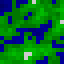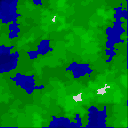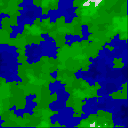# Play With Lua!

## Generating Heightmaps in Lua

Lua is a pretty much ideal language for playing around with map generating functions. I made a cute one today, combining linear noise with a couple other things.

To start off with, here’s how I create a Lua map class: I start by making a “Map” table that contains a “methods” table. Anything class-level (what Java would call “static”) goes in Map; anything instance-level goes is Map.methods. I make a Map.new function that creates a new Map of a certain size, filling it with either a constant value or linear noise (I’m using 16 elevation levels):

```Map = {methods={}}

-- Make a grid of random values 0..15, or a fill value
function Map.new(width, height, fill)
local map = {width=width, height=height}
setmetatable(map,{__index=Map.methods})

for n = 1, (width * height) do
map[n] = fill or (math.random(16) - 1)
end

return map
end```

Pretty basic so far. I’ll add a few methods like `at` and `set` that change values based on x and y coordinates.

One tricky thing: Lua libraries expect tables to be indexed from 1 (although it doesn’t really matter). So, I (probably inadvisedly) made mine like that, and then got plagued by off-by-one errors because I’ve written this class so many times in other languages that it’s practically muscle memory.

So anyway, I’m going to skip a lot of these functions and just describe what they do. I start off with a small map that I fill with noise, then run a simple smoothing function over. The smoothing function just takes, for each cell, the average of its value and the values of all its neighbors:

```function Map.methods.smooth(self)
local new = Map.copy(self)

for x = 0, self.width-1 do
for y = 0, self.height-1 do
local n = self:p2n{x=x,y=y}
local s, c = self:neighbor_sum(x, y)
local nv = (self:at(x,y) + s) / (c + 1)
new[n] = math.round(nv)
end
end

return new
end```

I keep this pattern of having every method return self so I can chain methods together. Then, I scale the map up 8x, by making an 8×8 tile for each pixel, with the corners being the adjacent pixels. So, the pixel at (0,0) becomes the upper-left corner of a tile that also has (1,0) at the upper right, (0,1) at the lower left, and so on.

Now I can fractalize it. For each tile I pick new pixels to go in the middle of each edge and in the center, so the middle of the left side will be either the top-left or bottom-left pixels, and so on. Now I can divide it into four new tiles and recurse (because the new tiles have values in each of their corners).

Once I recurse all the way to the bottom, I run the smoothing function one more time on the new map and then print it out. Making an image out of it is another little trick: rather than deal with finding an image-writing library and figuring out its API, since I’m just playing around, I write the image data by hand in XPM format. X Pixmap is a really simple image format, readable by the Gimp and Emacs, that was invented for storing X Windows icons, and is human-readable and trivial to write. Here’s an example:

```/* XPM */
static char * map_xpm[] = {
"16 16 16 1",
"0 c #000033",
"1 c #000044",
"2 c #000055",
. . . etc . . .
"45575569ca787679",
"3457679aa998977a",
"4557799889b889ab",
. . . etc . . .
}```

Becomes this:So, since I can chain everything together, after playing with it some, I ended up with this to create a map:

```math.randomseed(1) -- Or whatever...
m = Map.new(17, 17):smooth():scale(8):fractal_tile(8):smooth():slice(0,0,128,128)
file = io.open("map.xpm", "w")
file:write(m:xpm())```

(Since what I’m scaling are actually the spaces between cells, in order to get 16 tiles with corners I need a seed map 17×17)

So, enough chatter. Let’s look at a few random maps:Here’s the code. I think these came out much better than my last attempt at mapmaking. The elevations actually work; mountains slope gradually down into seas.

Written by randrews

May 16th, 2011 at 8:26 pm

Posted in Uncategorized